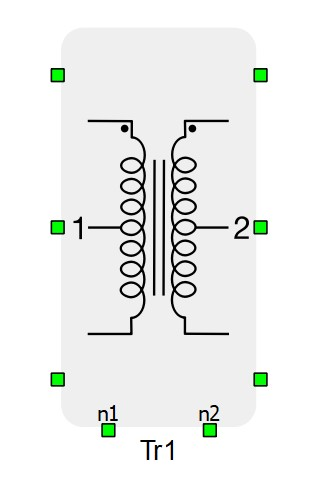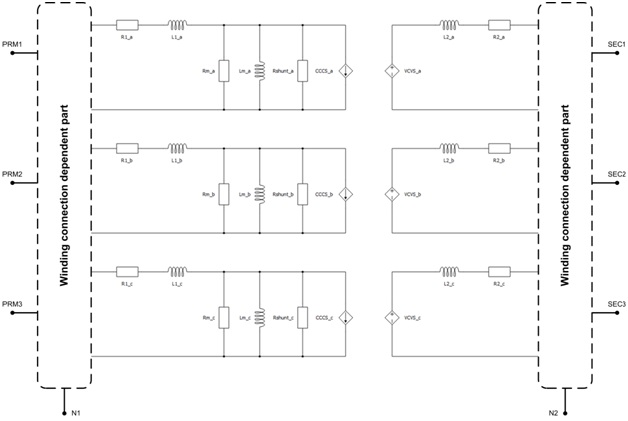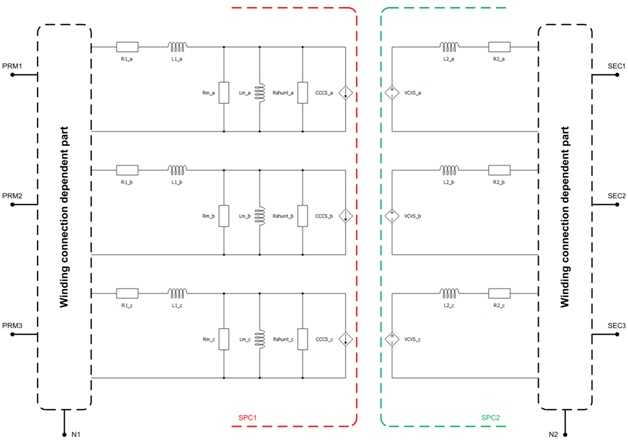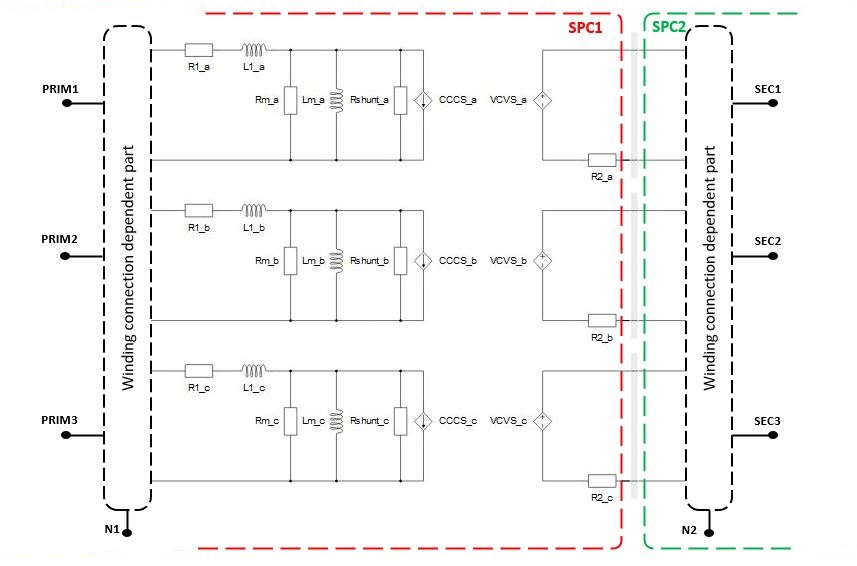# Three phase two winding transformer

This section describes three phase two winding transformer

The three-phase transformer is modeled as three single-phase transformers, meaning that only magnetic coupling between windings of the same phase are taken into account.

The magnetization inductance Lm can be linear or with saturation, it is modeled on the primary side of the transformer. Core losses are modeled as Rm resistance located on the primary side of the transformer. Also, it is possible to neglect Lm and Rm by selecting Lm/Rm neglected in the Core model property. For more informations, please refer to Core model

Schematic symbol and input parameters for three phase two winding transformer block are given in Table 1.

Table 1. Three phase two winding transformer component in HIL Toolbox
component component dialog window component parametersThree phase two winding transformer• Input parameters option (SC and OC tests, Si or p.u.)
• Nominal Power of the transformer (Sn)
• Nominal frequency (Hz)
• Nominal line to line voltage of the primary winding in volts RMS (V1)
• Nominal line to line voltage of the secondary winding in volts RMS (V2)
• Positive sequence short circuit voltage (% of nominal phase voltage) (usc1)
• Positive sequence short circuit losses (Psc1)
• Core model - Linear, Non-Linear, Rm/Lm neglected
• Positive sequence no load excitation current (% of nominal current) (ioc1)
• Positive sequence no load losses (Poc1)• Winding 1 connection (D or Y)
• Winding 2 connection (D or Y)
• Clock number - determines the phase displacement between primary and secondary side voltages• Embedded coupling - inserts a coupling component (Ideal Transformer based or TLM) in transformer.
• Type of coupling component - defines whether the inserted coupling component is a core or a device coupling. Device coupling is available only for the Ideal Transformer based coupling.
• TLM/Embedded components ratio - Defines how coupling to embedded inductors ratio will be calculated. Available only for the TLM coupling.
• Ratio - Used to specify coupling to embedded inductors ratio if Manual option is selected in TLM/Embedded components ratio property.
• Rshunt - resistor placed in parallel with the current source on the primary side. It is used to provide increase in model stability. Can be set to inf
There are two types of tests that are performed to characterize a transformer. Both of the tests are performed on either the primary or the secondary side of the transformer. The tests are:
• short circuit test – exciting a set of three-phase windings while the other set of windings is short circuited
• open circuit test – exciting a set of three-phase windings while the other set of windings is open circuited

Measurement results obtained from these tests and other information given on the transformer’s nameplate provide the necessary data for transformer characterization and modeling.

Winding excitation in the tests is three-phase positive sequence voltage. In addition to that, when characterizing a transformer and making a transformer model that includes mutual inductances between phases, it is necessary to perform the same tests, but with excitation being three-phase zero sequence voltage.

Parameters of the equivalent circuit are calculated as follows. During the short circuit test, the magnetization branch is considered shorted by the short circuited winding. So, primary side short circuit impedance is obtained:

${Z}_{sc}^{d}=3\frac{{u}_{sc}^{d}\left[%\right]{\left({V}_{1n}^{ph}\right)}^{2}}{{100S}_{n}}$

Primary side short circuit resistance is obtained:

${R}_{sc}=3{P}_{sc}^{d}{\left(\frac{{V}_{1n}^{ph}}{{S}_{n}}\right)}^{2}$

Primary side short circuit inductance:

${L}_{sc}^{d}=\frac{1}{2\pi {f}_{n}}\sqrt{{\left({Z}_{sc}^{d}\right)}^{2}-{\left({R}_{sc}\right)}^{2}}$

Primary and secondary side resistances and short circuit inductances are calculated using:

${R}_{1}=\frac{1}{2}{R}_{sc}$

${R}_{2}=\frac{1}{2}{R}_{sc}{\left(\frac{{N}_{2}}{{N}_{1}}\right)}^{2}$

${L}_{1}^{d}=\frac{1}{2}{L}_{sc}^{d}$

${L}_{2}^{d}=\frac{1}{2}{L}_{sc}^{d}{\left(\frac{{N}_{2}}{{N}_{1}}\right)}^{2}$

From the positive sequence open circuit test results, it is obtained:

${i}_{oc}^{d}=\frac{{i}_{oc}^{d}\left[%\right]}{100}{I}_{1n}^{ph}={i}_{oc}^{d}\left[%\right]\frac{{S}_{n}}{300{V}_{1n}^{ph}}$

${P}_{oc}^{d}=3\frac{{\left({V}_{1n}^{ph}\right)}^{2}}{{R}_{Fe}^{d}}\stackrel{yields}{\to }{R}_{Fe}^{d}=3\frac{{\left({V}_{1n}^{ph}\right)}^{2}}{{P}_{oc}^{d}}$

${P}_{oc}^{d}=3{R}_{Fe}^{d}{\left({i}_{Fe}^{d}\right)}^{2}\stackrel{yields}{\to }{\left({i}_{Fe}^{d}\right)}^{2}=\frac{{P}_{oc}^{d}}{3{R}_{Fe}^{d}}$

${i}_{m}^{d}=\sqrt{{\left({i}_{oc}^{d}\right)}^{2}-{\left({i}_{Fe}^{d}\right)}^{2}}$

${L}_{m}^{d}=\frac{1}{2\pi {f}_{n}}\frac{{V}_{1n}^{ph}}{{i}_{m}^{d}}$

Variables description:

Sn - nominal power of transformer

V1nph - primary side nominal phase to phase voltage

fn - nominal frequency

N2/N1 - transfer ration

uscd - short circuit voltage (sc) – positive sequence (d)

Zscd - short circuit impedance (sc) – positive sequence (d)

Pscd - short circuit active power (sc) – positive sequence (d)

Rsc - short circuit resistance (sc)

Lscd - short circuit inductance (sc) – positive sequence (d)

R1 - resistance on primary side

R2 - resistance on secondary side

L1d - leakage inductance on primary side – positive sequence (d)

L2d - leakage inductance on secondary side – positive sequence (d)

iocd - open circuit (oc) excitation current – positive sequence (d)

i1nph - nominal phase current

Pocd - open circuit (oc) losses– positive sequence (d)

RFed (Rm) - resistance representing the core losses under nominal voltage – positive sequence (d)

iFed - current due to core losses under nominal voltage – positive sequence (d)

imd - magnetizing current – positive sequence (d)

Lmd - magnetizing inductance – positive sequence (d)

A schematic block diagram of the three-phase two-winding transformer block with the corresponding component arrangement and naming is shown in Figure 1.Figure 1. Schematic block diagram of three phase two winding transformer with corresponding components naming

It should be noted that terminals N1 and N2 can be connected with the rest of the circuit in schematic editor only if the corresponding side is wye (Y) connected.

## Embedded coupling

There are two possible options for embedded coupling in the single phase two winding transformer, Ideal Transformer based coupling and TLM coupling.

If Embedded coupling is Ideal Transformer, Ideal Transformer based coupling will be placed between two windings of transformer.

In Figure 2 is given a visual representation of division of transformer's equivalent circuit in the case where Embedded coupling is set to Ideal Transformer.Figure 2. Representation of circuit division between cores where Embedded coupling is set to 'Ideal Transformer'

If Embedded coupling is set to TLM, secondary winding inductor in the each phase will be replaced with TLM coupling component. Inductance will be divided between coupling and embedded inductors (inductors will be hidden in the TLM). TLM to embedded inductors ratio can be determined by compiler, but also it can be specified explicitly. If Automatic option is selected, ratio will be determined by discretization method. If Manual option is selected, ratio can be explicitly set to meet user requirements. For more information on TLM couplings please refer to Core couplings - TLM.

In Figure 3 is given a schematic representation of transformer's equivalent circuit when Embedded coupling is set to TLM.Figure 3. Representation of circuit division between cores where Embedded coupling is set to 'TLM'

## Analog output signals from three phase two winding transformer

Internal variables of the transformer are available for observation at the analog outputs. Names of the variables available for observation have extensions _a, _b and _c added to their names. These extensions correspond to variables of sub circuits of phases a, b and c, respectively.

Analog output variable name Description
Lm_a_name Current of magnetizing inductance in phase A
Lm_b_name Current of magnetizing inductance in phase B
Lm_c_name Current of magnetizing inductance in phase C
L1_a_name Current of phase A leakage inductance on primary side
L1_b_name Current of phase B leakage inductance on primary side
L1_c_name Current of phase C leakage inductance on primary side
L2_a_name Current of phase A leakage inductance on secondary side
L2_b_name Current of phase B leakage inductance on secondary side
L2_c_name Current of phase C leakage inductance on secondary side